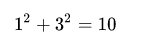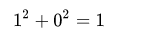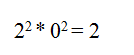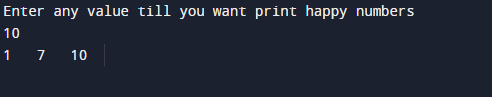C Program to print all Happy Numbers till N

In this tutorial you will learn about the C Program to print all Happy Numbers till N and its application with practical example.

In this tutorial, we will learn to create a C program that will find Happy number  using C programming.

Prerequisites

Before starting with this program  we assume that you are best aware of the following C programming topics:

• C Operators.
• C loop statements.
• C while and for Loop.
• Conditional statement.

What Is Happy Number ?

Happy number is a number which finally ends at 1 when it is replaced by the sum of the square of its digits repeatedly.

Example

let’s take an example here 13 is a happy number,   and.

On the other hand,The unhappy number will result in a cycle of 4, 16, 37, ….

2 is not a happy number let’s have a look an example of thisand  this process continues to infinite cycle without ever reaching 1

C Program to print all Happy Numbers till N

In this program we will find that given number is happy number or not using for loop.
Firstly we declare required header file and variable and also initiates required values in variable. Next we take value from user at run time and then after we will find that the given value is happy number or not.

Outputhappy number is defined by the following steps:

• STEP 1: START.
• STEP 2: SET tmp =0, sum =0.
• STEP 3: REPEAT STEP 4 to 6 UNTIL (no>0)
• STEP 4: tmp=no%10.
• STEP 5: sum = sum + (tmp*tmp)
• STEP 6: no= no/10.
• STEP 7: RETURN sum.
• STEP 8: END.
• If the number given is greater than 0 (no>0 ),First we find remainder by dividing the number with 10  ( tmp=no %10 ).
• Calculate square of tmp and add it to sum.
• Then divide number by 10 ( no=no/10).
• Repeat the steps from 4 to 6 until the sum of the square of all digits present in number has been calculated.
• Finally, print  the sum.

So finally we get all the happy number from one to given number by user…..

In this tutorial we have learn about the C Program to print all Happy Numbers till N and its application with practical example. I hope you will like this tutorial.